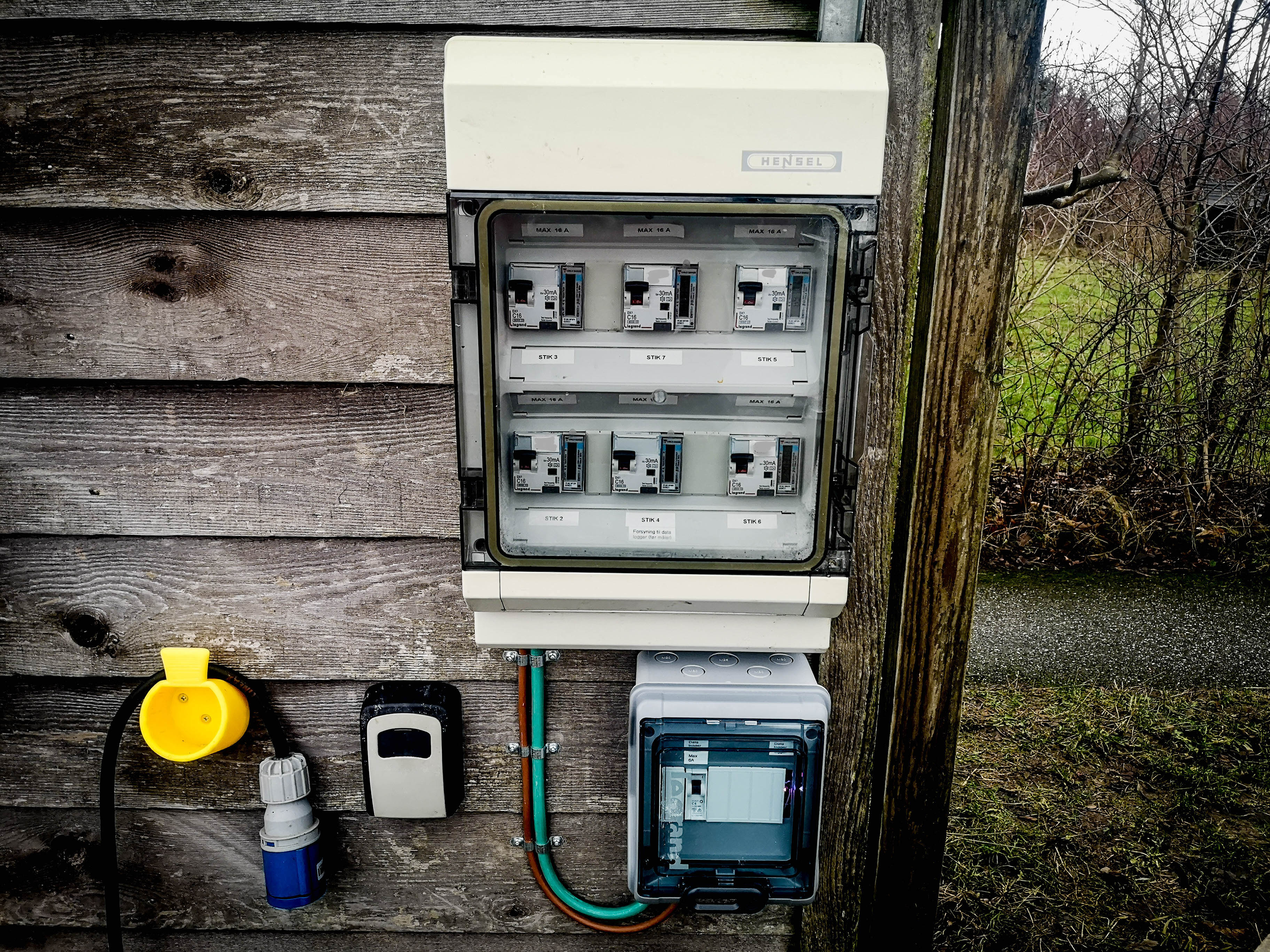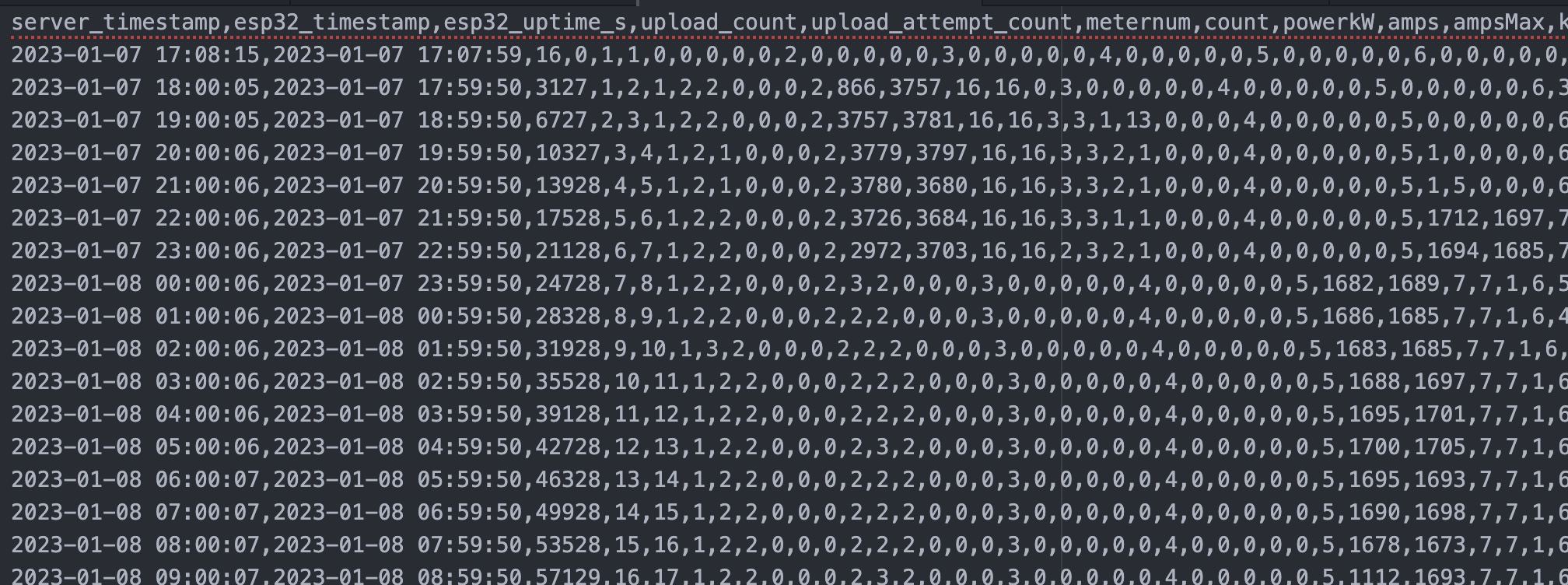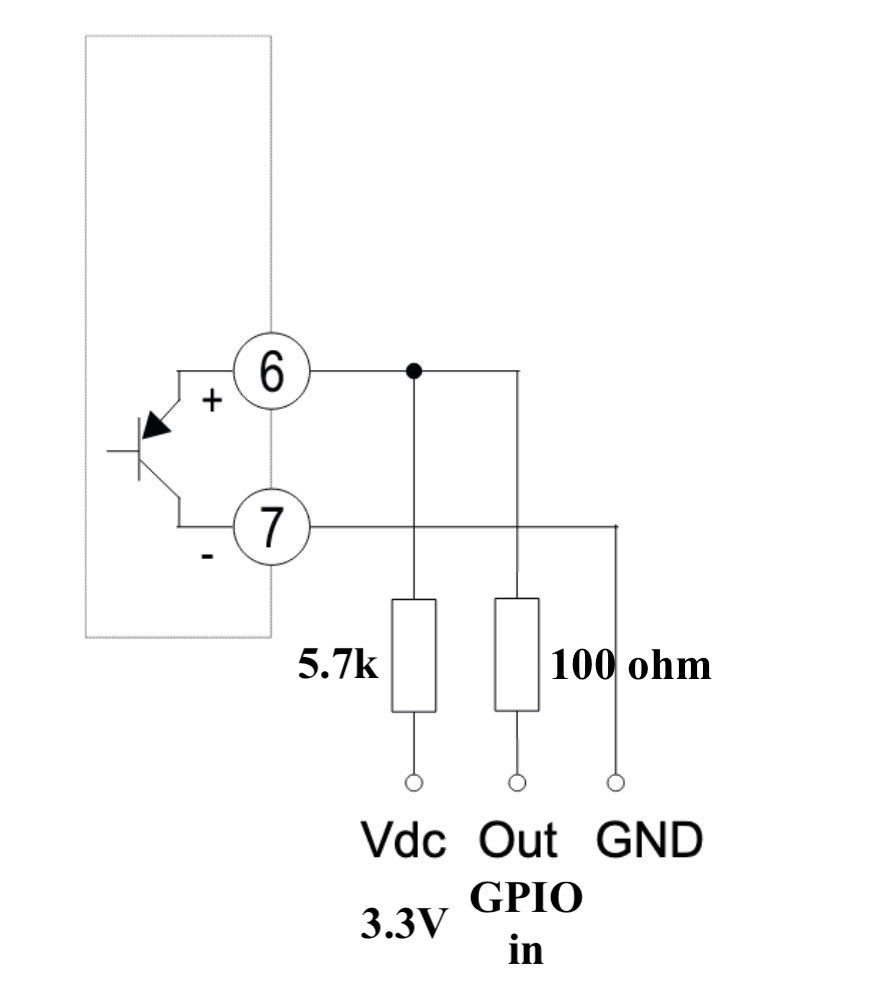Published: January 09, 2023, Edited by: Nicolas Padfield

# Logging multiple energy meters with one ESP32 and getting NORDPOOL electricity prices

An after hours open sourced project by Nicolas, here is how to use one cheap ESP32 (DKR35/USD5/EUR5) to

• Log the realtime electricity consumption on a standard electricity meter, using the standard pulse output
• Log multiple meters (here 6, but up to about 30 should be possible, limited only by the number of free GPIO pins)
• Upload the data to a server
• Get the current electricity price in Denmark/Scandinavia/Nordpool
• Calculate the total electricity price in Denmark, consisting of energinet, nettarif, elafgift, elspot, VAT. For example, currently (in DKR):
• Energinet: 0.14
• Nettarif: 1.9135
• Elspot: 1.34862503
• Total: 3.41212503

The energy meters can be any industry standard energy meter with a pulse output. In this case DIN rail Carlo Gavazzi EM111 (PDF datasheet). These are single phase 230V 45A meters, but the advantage of using pulse reading is that almost all meters support it, including huge 3 phase current transformer 400A meters.

In Denmark at the moment, it is not unusual for the electricity price to be 0.4 kr/kWh at night and 5 kr/kWh during the 1700-2100 peak hours - a factor 15 difference! This project is to enable correct billing of multiple electric cars charging from a communal solution, without needing an expensive internet connected EVSE charger or smart meter per car.The 6 meters and data logger enclosure.The CSV file generated on the server.

When connecting pulse outputs on meters, remember

• Only qualified personnel!
• Double insulation
• Insulated end terminals make it a lot easier to make nice and safe

How to connect the open collector pulse output on the electricity meter to a microcontroller:I use a 5.7k pullup resistor. You can try using the 20k built in pullup resistor, but that is quite a high value at 3.3V if the cable is long.
The 100 ohm resistor is to protect the microcontrollers input. It will work without, but may not continue working in a real world setting and with long wires.

The ESP32 code uses a timer interrupt to sample the input pins 1000 times a second.

The meter pulses are 30ms or 100ms. 60ms is also common. With sampling at 1000 Hz, we can be sure to get all pulses, and at a high enough resolution to calclulate the approximate energy (kW) now. This is however only used to calculate the current per phase, not for billing. For billing, the counted number of 1 Wh pulses is the most accurate measure.

#### ESP32 code:

``````// https://www.energidataservice.dk/tso-electricity/DatahubPricelist
// https://api.energidataservice.dk/dataset/Elspotprices?limit=100&offset=0&start=2022-08-22T00:00&end=2022-08-22T00:00&filter=%7B%22PriceArea%22:%22DK2%22%7D&sort=HourUTC%20DESC&timezone=utc
// https://www.energidataservice.dk/guides/api-guides
// https://www.energidataservice.dk/tso-electricity/Elspotprices
// https://api.energidataservice.dk/dataset/Elspotprices?start=2022-08-18T05:00&end=2022-08-18T06:00&filter={%22PriceArea%22:%22dk2%22}

// https://cplusplus.com/reference/ctime/strftime/

/*

Common gnd to one side of electricity meter pulse output.
Wire to other side of electricity meter pulse output.
100 ohm in series from wire before it gets to esp32 for protection
5.7k pullup from each to +3.3V (I don't trust the built in 20K pullup to be enough when these are long wires)

*/

#include <WiFi.h>
#include "time.h"
#include "sntp.h"
#include "credentials.h"
// esp_crt_bundle.h
#include <HTTPClient.h>

/*

// Credentials should be defined in a file credentials.h like this:

#define WIFIPASS "..."
#define WIFISSID "..."

*/

// uint32_t / float

boolean led = false;
boolean newlyBooted = true;
unsigned long uploadInterval = 3600;  // in seconds, normally 3600 for one hour, except for testing
unsigned long rebootAfter = 5400000;  // ms reboot if no succesfull uploads for 1.5 hours
unsigned long previousWifiReconnectMillis = 0;
unsigned long wifiReconnectInterval = 30000;
unsigned long statusTime = 0;
unsigned long statusTimeInterval = 2000;

char timeStringBuff;  //50 chars should be enough

String serverName = "https://.../getprice.php";
String serverPath = "";
#define ALLOCATION_SIZE 512  // size to allocate for serverPath string - attempt to avoid fragmentation

hw_timer_t* My_timer = NULL;

const char* ntpServer1 = "pool.ntp.org";
const char* ntpServer2 = "time.nist.gov";
const long gmtOffset_sec = 3600;
const int daylightOffset_sec = 3600;

boolean inputNow = { 0, 0, 0, 0, 0, 0 };
boolean inputLast = { 0, 0, 0, 0, 0, 0 };

unsigned long pulseTime = { 0, 0, 0, 0, 0, 0 };
unsigned long lastTime = { 0, 0, 0, 0, 0, 0 };

unsigned long count = { 0, 0, 0, 0, 0, 0 };
float powerkW = { 0, 0, 0, 0, 0, 0 };
float amps = { 0, 0, 0, 0, 0, 0 };
float ampsMax = { 0, 0, 0, 0, 0, 0 };
float kWh = { 0, 0, 0, 0, 0, 0 };

unsigned int ppwh = 1;

const char* time_zone = "CET-1CEST,M3.5.0,M10.5.0/3";  // TimeZone rule for Europe/Rome including daylight adjustment rules (optional)

// 1672534790 = Sun Jan 01 2023 00:59:50 GMT+0000

if ((getUnixTime() - 1672534790) % uploadInterval == 0) {  // uploadInterval should normally be 3600, except during testing
// it is x:59:50 of some hour
return true;
} else {
return false;
}
}

// Function that gets current epoch time
unsigned long getUnixTime() {
time_t now;
struct tm timeinfo;
if (!getLocalTime(&timeinfo)) {
//Serial.println("Failed to obtain time");
return (0);
}
time(&now);
return now;
}

void getCharLocalTime() {
time_t rawtime;
struct tm timeinfo;
if (!getLocalTime(&timeinfo)) {
Serial.println("Failed to obtain time");
return;
}

strftime(timeStringBuff, sizeof(timeStringBuff), "%Y-%m-%d+%H:%M:%S", &timeinfo);
}

void printLocalTime() {
struct tm timeinfo;
if (!getLocalTime(&timeinfo)) {
Serial.println("No time available (yet)");
return;
}
Serial.println(&timeinfo, "%A, %B %d %Y %H:%M:%S");
}

// Callback function (gets called when time adjusts via NTP)
void timeavailable(struct timeval* t) {
printLocalTime();
}

void IRAM_ATTR onTimer() {
// do stuff

for (int i = 0; i < 6; i++) {

if (!inputNow[i] && inputLast[i]) {  // there was a pulse leading edge

//used to measure time between pulses.
lastTime[i] = pulseTime[i];
pulseTime[i] = micros();

//Serial.print(" edge ");
count[i]++;

//Calculate power
powerkW[i] = (3600000000.0 / (pulseTime[i] - lastTime[i])) / ppwh;

// Calculate current
amps[i] = powerkW[i] / 230.0;

if (amps[i] > ampsMax[i]) {
ampsMax[i] = amps[i];
}

//Find kwh elapsed
kWh[i] = (1.0 * count[i] / (ppwh * 1000));  //multiply by 1000 to convert pulses per wh to kwh
}
inputLast[i] = inputNow[i];
}
}

void setup() {

pinMode(25, INPUT);  // 1
pinMode(27, INPUT);  // 2
pinMode(32, INPUT);  // 3
pinMode(33, INPUT);  // 4
pinMode(34, INPUT);  // 5
pinMode(35, INPUT);  // 6

pinMode(LED_BUILTIN, OUTPUT);

serverPath.reserve(ALLOCATION_SIZE);  // pre-allocate string memory

Serial.begin(115200);

My_timer = timerBegin(0, 80, true);
timerAttachInterrupt(My_timer, &onTimer, true);
timerAlarmWrite(My_timer, 1000, true);  // 1000000 microseconds = 1 hz. 1000 = 1000 hz
timerAlarmEnable(My_timer);             //Just Enable

/**
* NTP server address could be aquired via DHCP,
*
* NOTE: This call should be made BEFORE esp32 aquires IP address via DHCP,
* otherwise SNTP option 42 would be rejected by default.
* NOTE: configTime() function call if made AFTER DHCP-client run
* will OVERRIDE aquired NTP server address
*/
sntp_servermode_dhcp(1);  // (optional)

/**
* This will set configured ntp servers and constant TimeZone/daylightOffset
* should be OK if your time zone does not need to adjust daylightOffset twice a year,
* in such a case time adjustment won't be handled automagicaly.
*/
configTime(gmtOffset_sec, daylightOffset_sec, ntpServer1, ntpServer2);

/**
* A more convenient approach to handle TimeZones with daylightOffset
* would be to specify a environmnet variable with TimeZone definition including daylight adjustmnet rules.
* A list of rules for your zone could be obtained from https://github.com/esp8266/Arduino/blob/master/cores/esp8266/TZ.h
*/
//configTzTime(time_zone, ntpServer1, ntpServer2);

//connect to WiFi
Serial.printf("Connecting to %s ", WIFISSID);
WiFi.begin(WIFISSID, WIFIPASS);
while (WiFi.status() != WL_CONNECTED) {
delay(500);
Serial.print(".");
}
Serial.println(" CONNECTED");
}

void loop() {

if (millis() - statusTime > statusTimeInterval) {
statusTime = millis();

Serial.print("Counts");

for (int i = 0; i < 6; i++) {
Serial.print(" ");
Serial.print(count[i]);
}
Serial.print(" ");
printLocalTime();  // it will take some time to sync time :)

if (WiFi.status() == WL_CONNECTED) {
digitalWrite(LED_BUILTIN, led);
led = !led;
}
}

unsigned long currentMillis = millis();
// if WiFi is down, try reconnecting every interval seconds
if ((WiFi.status() != WL_CONNECTED) && (currentMillis - previousWifiReconnectMillis >= wifiReconnectInterval)) {
Serial.print(millis());
Serial.println("Reconnecting to WiFi...");
WiFi.disconnect();
WiFi.reconnect();
previousWifiReconnectMillis = currentMillis;
}

//Check WiFi connection status
if (WiFi.status() == WL_CONNECTED) {
HTTPClient http;

getCharLocalTime();  // update time

serverPath = serverName;
serverPath += "?p=";
serverPath += "&d=";
serverPath += String(timeStringBuff);
serverPath += ",";
serverPath += millis() / 1000;  // uptime
serverPath += ",";
serverPath += ",";
serverPath += ",";

for (int i = 0; i < 6; i++) {
serverPath += i + 1;
serverPath += ",";
serverPath += count[i];
serverPath += ",";
serverPath += powerkW[i];
serverPath += ",";
serverPath += amps[i];
serverPath += ",";
serverPath += ampsMax[i];
serverPath += ",";
serverPath += kWh[i];
serverPath += ",";

// clear some things
count[i] = 0;
powerkW[i] = 0;
amps[i] = 0;
ampsMax[i] = 0;
kWh[i] = 0;
}

Serial.print("serverPath: ");
Serial.println(serverPath);

// Your Domain name with URL path or IP address with path
http.begin(serverPath.c_str());

// If you need Node-RED/server authentication, insert user and password below

// Send HTTP GET request
int httpResponseCode = http.GET();

if (httpResponseCode > 0) {
Serial.print("HTTP Response code: ");
Serial.println(httpResponseCode);
} else {
Serial.println(httpResponseCode);
}
// Free resources
http.end();

newlyBooted = false;

} else {
}
}

if (millis() - lastTimeUploaded > rebootAfter) {
ESP.restart();
}
}
``````

#### PHP code, on server end:

``````<?php

\$pass = "...";
\$iAmReallyInDenmarkAndWantToGetPrice = false; // change to true if you really want to bother the API server

// https://www.energidataservice.dk/tso-electricity/DatahubPricelist
// https://www.energidataservice.dk/guides/api-guides
// https://www.energidataservice.dk/tso-electricity/Elspotprices

\$startTime = gmdate("Y-m-d\TH:i", time()-3660);
\$endTime = gmdate("Y-m-d\TH:i", time()-60);

//\$url = "https://api.energidataservice.dk/dataset/Elspotprices?limit=10&offset=0&start=2022-08-22T00:00&end=2022-08-22T00:00&filter=%7B%22PriceArea%22:%22DK2%22%7D&sort=HourUTC%20DESC&timezone=utc";
\$url = "https://api.energidataservice.dk/dataset/Elspotprices?limit=10&offset=0&start=\$startTime&end=\$endTime&filter=%7B%22PriceArea%22:%22DK2%22%7D&sort=HourUTC%20DESC&timezone=utc";

//echo \$url;
if(\$iAmReallyInDenmarkAndWantToGetPrice == true){
\$json = file_get_contents(\$url);
}
//echo \$json;

\$obj = json_decode(\$json);

//var_dump(\$obj);

\$elSpotExMoms = (\$obj->records->SpotPriceDKK)/1000.0;

//echo \$elSpotExMoms;

\$energinet = 0.140;
\$nettarif = getNetTarif(time());
\$elSpot = \$elSpotExMoms * 1.25;

\$ialt = \$energinet + \$nettarif + \$elafgift + \$elSpot;

if(!isset(\$_GET['p'])){
}
//\$d = strtotime('2023-08-01 20:00');
//echo "<br>";
//echo getNetTarif(\$d);

if (\$_GET['p'] == \$pass && strlen(\$_GET['d']) < 512 && strlen(\$_GET['d']) > 8) {

\$d = filter_var(\$_GET['d'],FILTER_SANITIZE_STRING);
\$d = urldecode(\$d);

\$fp = fopen('d.csv','a'); //opens file in append mode

\$r = fwrite(\$fp, date("Y-m-d H:i:s") . "," . \$d . "". \$energinet . ",".  \$nettarif . ",".  \$elafgift . ",".  \$elSpot . ",". \$ialt . "\n");
fclose(\$fp);
if(\$r){
echo "ok";
}
else{
echo "error";
}

}

\$r=2000000000;

if(date('Y', \$d)== 2023 && date('m', \$d)<=6){ // it is first half of 2023
\$r=0.01;
}
else {
\$r=0.904;
}
return \$r;
}

function getNetTarif(\$d){
\$r=1000000000;

if(date('Y', \$d)== 2023 && date('m', \$d)==1){
// until 1. feb 2023
if(date('m', \$d)>=10 || date('m', \$d)<=3){ // it is winter

if(date('H', \$d)>=0 && date('H', \$d)<=5){ // it is lavlast
\$r = 21.27;
}
else if(date('H', \$d)>=17 && date('H', \$d)<=20){ // it is spidslast
\$r = 191.35;
}
else { // it must be højlast
\$r=63.79;
}
}
else { // it is summer

if(date('H', \$d)>=0 && date('H', \$d)<=5){ // it is lavlast
\$r = 21.27;
}
else if(date('H', \$d)>=17 && date('H', \$d)<=20){ // it is spidslast
\$r = 82.92;
}
else { // it must be højlast
\$r=31.89;
}
}
// until 1. feb 2023
}

if(date('Y', \$d)== 2023 && date('m', \$d)==2){
// 1. feb to 1. marts 2023
if(date('m', \$d)>=10 || date('m', \$d)<=3){ // it is winter

if(date('H', \$d)>=0 && date('H', \$d)<=5){ // it is lavlast
\$r = 18.37;
}
else if(date('H', \$d)>=17 && date('H', \$d)<=20){ // it is spidslast
\$r = 165.33;
}
else { // it must be højlast
\$r=55.11;
}
}
else { // it is summer

if(date('H', \$d)>=0 && date('H', \$d)<=5){ // it is lavlast
\$r = 18.37;
}
else if(date('H', \$d)>=17 && date('H', \$d)<=20){ // it is spidslast
\$r = 71.64;
}
else { // it must be højlast
\$r=27.56;
}
}
// 1. feb to 1. marts 2023
}

if((date('Y', \$d)== 2023 && date('m', \$d)>2) || date('Y', \$d)> 2023){

// from 1. marts 2023
if(date('m', \$d)>=10 || date('m', \$d)<=3){ // it is winter

if(date('H', \$d)>=0 && date('H', \$d)<=5){ // it is lavlast
\$r = 15.09;
}
else if(date('H', \$d)>=17 && date('H', \$d)<=20){ // it is spidslast
\$r = 135.84;
}
else { // it must be højlast
\$r=45.28;
}
}
else { // it is summer

if(date('H', \$d)>=0 && date('H', \$d)<=5){ // it is lavlast
\$r = 15.09;
}
else if(date('H', \$d)>=17 && date('H', \$d)<=20){ // it is spidslast
\$r = 58.87;
}
else { // it must be højlast
\$r=22.67;
}
}
// from 1. marts 2023
}

return \$r/100;
}

?>
``````